The circle formulas below are a convenient reference for many of the commonly used formulas for Amateur Radio. They way they work is fairly simple. Cover up the answer you are looking for and what you will be left with is the formula to calculate it.

Below is an excerpt from http://arfunk.com/fun/amateur-ham-radio/guide-to-passing-amateur.html which provides an example of using the circle formula for Frequency.

There is an easy to remember relationship between frequency and wavelength:

Frequency in MHz x Wavelength in Meters = 300

But who wants to remember a formula and use algebra to turn it into the form needed for a given problem? Nope, there’s an easier way to remember, and use, this formula. Look at this circle: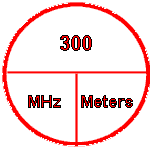Frequency – Wavelength Circle

Not only is it easy to remember, it’s easy to use. Say you have the wavelength in meters and need to find the frequency. Using the diagram, put your hand over what you are looking for, in this case the frequency in MHz. What’s left? 300/meters. Divide 300 by the wavelength in meters and you will get the frequency in MHz.

If you have the frequency in MHz and are looking for the wavelength, cover meters in the circle and you’ll have 300/MHz left. Divide 300 by the frequency in MHz and you’ll get the wavelength in meters.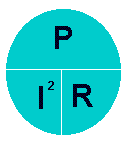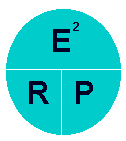### Power

E=Voltage in Volts
I=Current in Amps
R=Resistance in Ohms
P=Power in Watts
I=Current in Amps
E=Voltage in Volts
E=Voltage in Volts
R=Resistance in Ohms
P=Power in Watts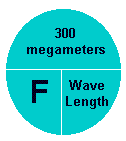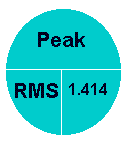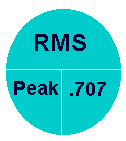### AC Voltage

R=Resistance in Ohms
G=Conductance in Mhos
F=Frequency in Hertz
Wavelength in Meters
RMS=Root Mean Square value
Peak=Peak voltage in a sine wave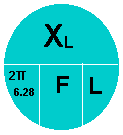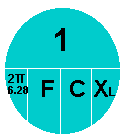### Capacitive Reactance

T=Time in Seconds
R=Resistance in Ohms
T=Time in Seconds
F=Frequency in Hertz
Xl=Inductive Reactance in Ohms
F=Frequency in Hertz
L=Impedance in Henrys
F=Frequency in Hertz
Xc=Capacitive Reactance in Ohms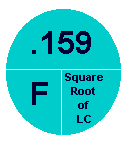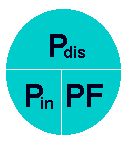## Efficiency

En=Energy
P=Power in Watts
T=Time in Seconds
F=Frequency in Hertz Pdis=Power Dissipated in Watts
Pin=Power in in Watts
PF=Power Factor
Pout=Power out in Watts
Pin=Power in in Watts
Eff=Efficiency